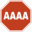# AAAAAAAAA! (language)

(Redirected from AAAAAAAA! (language))AAAA!This page contains a strong encryption algorithm. Import to countries such as Iran, Cuba, and North Korea may be illegal.

“This is SpaghetAAAAAAAAA!”

~ LeonidAAAAAAAAs on In Italic Restaurant.

“AaAaAaAaAaAaA!”

~ Homsar on AAAAAAAA!

“NNNNNNNNN!”

~ EBG13 on AAAAAAAA!

AAAAAAAAA! is a special code used by space pirates, zombies, and people like you in order to confuse anyone who might tap into their phone lines. [Aaaaaaaa aaa aaaaaa aa aaa, aaa AAAA!]

AAAAAAAAA! has some very unique properties:

• Extremely fast encryption.
• The algorithm is symmetric: encryption and decryption take equally long.
• Easy to pronounce by speakers of nearly any language (compare to gibberish generated by ciphers, eg LK AH IO FU KK IT KJ HE).
• Can be encrypted easily even on an 8 bit machine if the characters involved are ASCII, 16 bit if they're Unicode.
• No encryption keys to keep safe & worry about.
• Very nearly almost unbreakable.

## Example of translating into AAAAAAAAA!

First assign to every character a number that corresponds to its place in the character set, like so for the English alphabet:

```A B C D E F G H I J  K  L  M  N  O  P  Q  R  S  T  U  V  W  X  Y  Z
1 2 3 4 5 6 7 8 9 10 11 12 13 14 15 16 17 18 19 20 21 22 23 24 25 26
```

Now subtract 13.5 (ie, half of the number of characters + 0.5) from each number. We now have:

```  A     B     C    D    E    F    G    H    I    J    K    L    M    N   O   P   Q   R   S   T   U   V   W   X    Y    Z
-12.5 -11.5 -10.5 -9.5 -8.5 -7.5 -6.5 -5.5 -4.5 -3.5 -2.5 -1.5 -0.5 0.5 1.5 2.5 3.5 4.5 5.5 6.5 7.5 8.5 9.5 10.5 11.5 12.5
```

Now, take the absolute value of the first character, and divide by the absolute value of the last character. Repeat with the second character, and the second to last character, all the way through the character set.

```12.5/12.5 = 1
11.5/11.5 = 1
10.5/10.5 = 1
9.5/9.5 = 1
8.5/8.5 = 1
7.5/7.5 = 1
6.5/6.5 = 1
5.5/5.5 = 1
4.5/4.5 = 1
3.5/3.5 = 1
2.5/2.5 = 1
1.5/1.5 = 1
0.5/0.5 = 1
0.5/0.5 = 1
1.5/1.5 = 1
2.5/2.5 = 1
3.5/3.5 = 1
4.5/4.5 = 1
5.5/5.5 = 1
6.5/6.5 = 1
7.5/7.5 = 1
8.5/8.5 = 1
9.5/9.5 = 1
10.5/10.5 = 1
11.5/11.5 = 1
12.5/12.5 = 1
```

Now, replace the numeric values with the corresponding character (1 = A, 2 = B, etc.):

```1 = A
1 = A
1 = A
1 = A
1 = A
1 = A
1 = A
1 = A
1 = A
1 = A
1 = A
1 = A
1 = A
1 = A
1 = A
1 = A
1 = A
1 = A
1 = A
1 = A
1 = A
1 = A
1 = A
1 = A
1 = A
1 = A
```

Now, replace your unencrypted letters with their encrypted values, and place an exclamation mark at the end. This is called salting.

```I have to go now = A AAAA AA AA AAA!
Mommy, let's go to the store = AAAAA, AAA'A AA AA AAA AAAAA!
```
```Make your time = AAAA AAAA AAAA!
```
```The chopper is down! = AAA AAAAAAA AA AAAA!!
```
```AAAAAA AAA AAAAA! = AAAAAA AAA AAAAA!!
```

Type the following code to your address bar then you can encrypt AAAAAAAAA!

``` javascript:prompt("AAAA AAA AAAAAAAAA AAAAAA:", (prompt("AAAAA AAAA AAAAAA!")).replace(/[A-Za-z0-9]/g,"A") ); void 0;
```

## Guide to translating from AAAAAAAAA!

This cipher is probably a linear one-to-one mapping (ie, it can be represented by a matrix with a non-zero determinant), therefore the steps must be followed backwards in order to decrypt the code. This makes it difficult, if not impossible, for unwanted parties to retrieve the encrypted information.

AAAAAAAAAAAAAAAAAAAAAAAAAAAAAAAAAAAAAAAAAAAAAAAAAAAAAAAAAAAAAAAAAAAAAAAAAAAAAAAAAAAAAAAAAAAA! tails